Latest Banking jobs   »   Quantitative Aptitude Quiz For SBI Clerk...

# Quantitative Aptitude Quiz For SBI Clerk Mains 2022- 21th December

Directions (1-5): Read the given information carefully and answer the following questions.

Arun & Veer invested Rs. 60,000 and Rs. 90,000 respectively. Arun invested for 15 months while Veer invested for 8 months and the difference between profit share of Arun and Veer is Rs.10000. Arun invested his profit share at 20% p.a on CI for 1.5 years and he bought a bike from the amount he received. Veer bought two laptops L₁ & L₂ (both laptops have equal price) from the amount which he received after investing his profit share at 15% p.a on SI for 2 years. Veer sold L₁ & L₂ at 10% and 15% profit respectively while Arun sold his bike at 5% loss.

Q1. Veer invested money (which he gets from the profit of that two laptops) in a scheme at the rate of 10% p.a on simple interest for 4 years. What is the interest he received?
(a) Rs. 2800
(b) Rs. 2600
(c) Rs. 2500
(d) Rs. 2750
(e) Rs. 3000

Q2. If the price of Bike depreciates 15% every year, then what will be the price of bike after 2 years?
(a) Rs. 47,685
(b) Rs. 49,675
(c) Rs. 48,025
(d) Rs. 47,515
(e) Rs. 48,195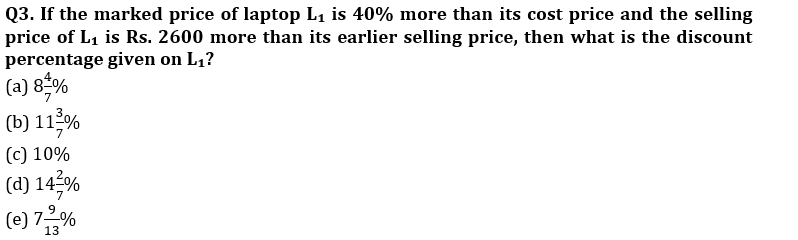Q4. The total amount received by Arun on selling that bike and the total amount received by veer on selling both the laptops together is how much more or less than the 108% of total cost price of all the three items together?
(a) Rs. 6040
(b) Rs. 6180
(c) Rs. 6240
(d) Rs. 6340
(e) Rs. 6380

Q5. In the above passage, if the difference between profit share of Arun and Veer is Rs. 11000 instead of Rs. 10000, then what will be the change in the net profit earned on all the three items together? (Rest all information remains same)
(a) Rs. 120
(b) Rs. 320
(c) Rs. 220
(d) Rs. 520
(e) Rs. 420

Directions (6-10): Study the bar charts given below and answer the following questions.
Bar chart shows the units of soaps manufactured by two different companies (A & B) in 5 different years and percentage of soaps sold by these 2 companies in these 5 years. Both companies started their production from 2014.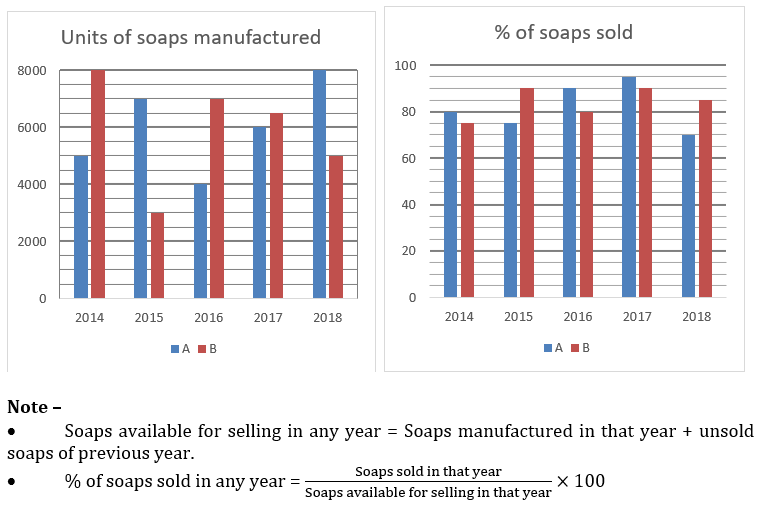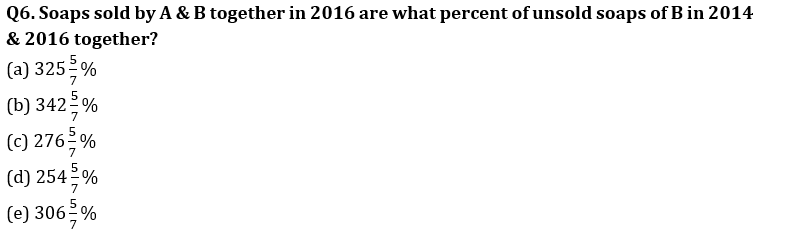Q7. Average of unsold units of B in all 5 years is how much more or less than unsold units of A in 2016 & 2017 together?
(a) 244
(b) 282
(c) 268
(d) 204
(e) 238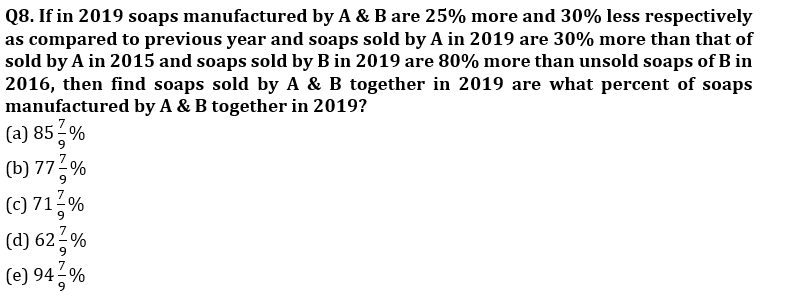Q9. If selling price of each soap of A & B in all years is Rs.13 & Rs.17 respectively, then find revenue of A in 2016, 2017 & 2018 together is how much more or less than revenue of B in 2015, 2016 & 2017 together?
(a) Rs.85,478
(b) Rs.73,387
(c) Rs.61,792
(d) Rs.68,456
(e) Rs.79,889

Q10. If cost price and selling price of each unit of soap of B in all the given years is Rs.15 & Rs.15.80 respectively and B threw all its unsold units of soaps in 2018 as B closed down in 2018, then find approximate profit/loss percentage of B over all the 5 given years together.
(a) 8%
(b) 10%
(c) 13%
(d) 7%
(e) 2%

Anurag invested Rs. (P) at the rate of (R)% for four years and get Rs. 600 as interest. Anurag by investing all the amount he received in a business with Ayush, who invested capital of Rs. 64R (Ratio for time period for Anurag & Ayush invested is 3 : 4) and Ayush gets total Rs. 3200 as profit share out of total profit of Rs. 8600. Veer sold an article at Rs. 72R after allowing a discount of (d)% on its marked price (M), instead of (M), if allowed same discount on (P), then he sold the article at 80R and made a profit of 33⅓%.

Q11. Find the value of (12R + 3P)?
(a) 4950
(b) 4450
(c) 2750
(d) 3250
(e) 3750

Q12. Find value of d% of (566 + 1234)?
(a)1 300
(b) 200
(c) 250
(d) 275
(e) 240

Q13. In the given question, two quantities are given, one as ‘Quantity I’ and another as ‘Quantity II’. You have to determine relationship between two quantities and choose the appropriate option:
Quantity I – 300% of M
Quantity II – 270% of P
(a) Quantity I ≥ Quantity II
(b) Quantity I = Quantity II or No relation
(c) Quantity I > Quantity II
(d) Quantity I < Quantity II
(e) Quantity I ≤ Quantity II

Q14. Find the value of M/R ?
(a) 84.5
(b) 88.5
(c) 86.4
(d) 90.6
(e) 80.4

Q15. If selling price of an article is Rs. (M + P) after allowing two successive discounts on Rs. 3800 of ‘X’% and (X – 5)%, then find value of 2X? (some profit is earned on selling)
(a) 40
(b) 50
(c) 25
(d) 30
(e) None of these

Solutions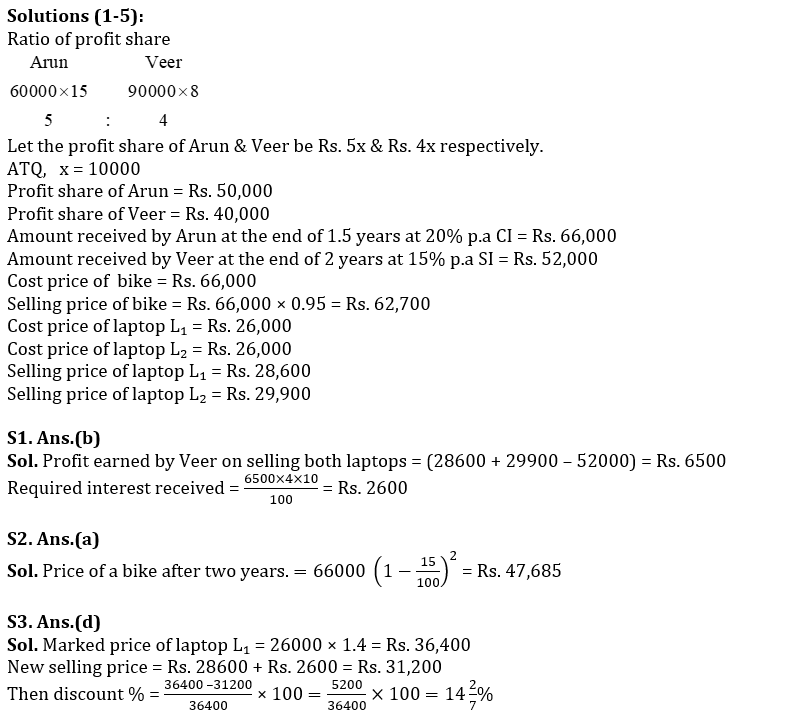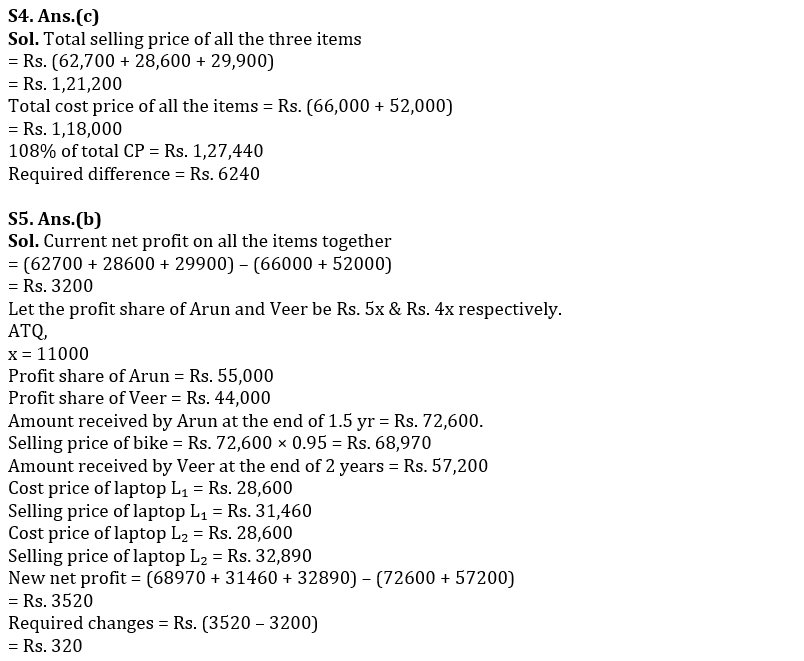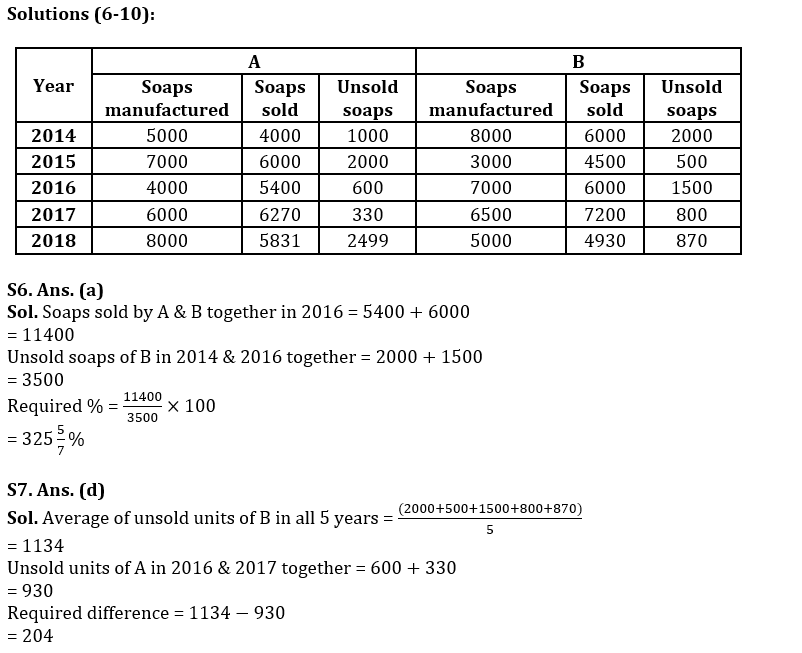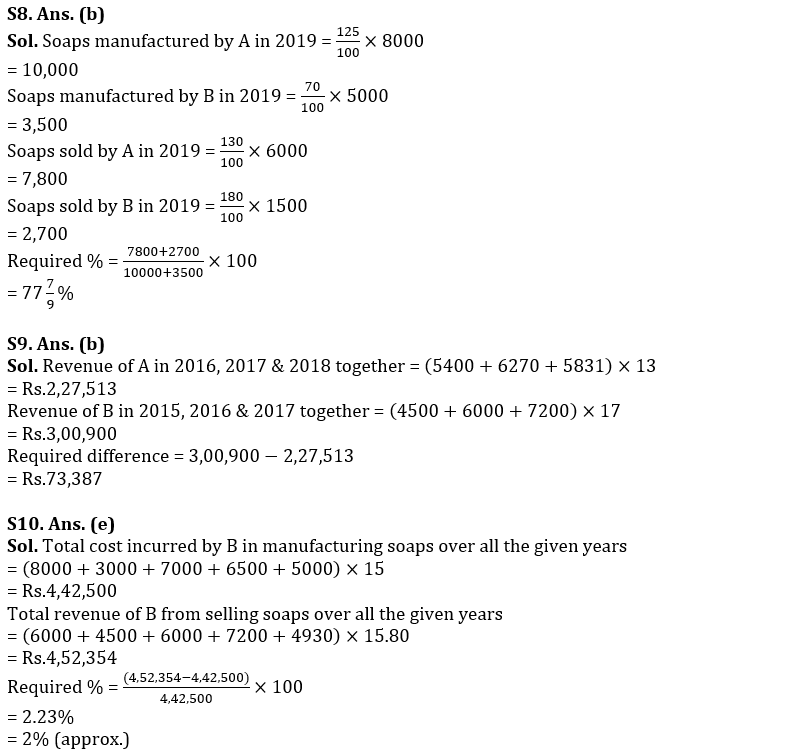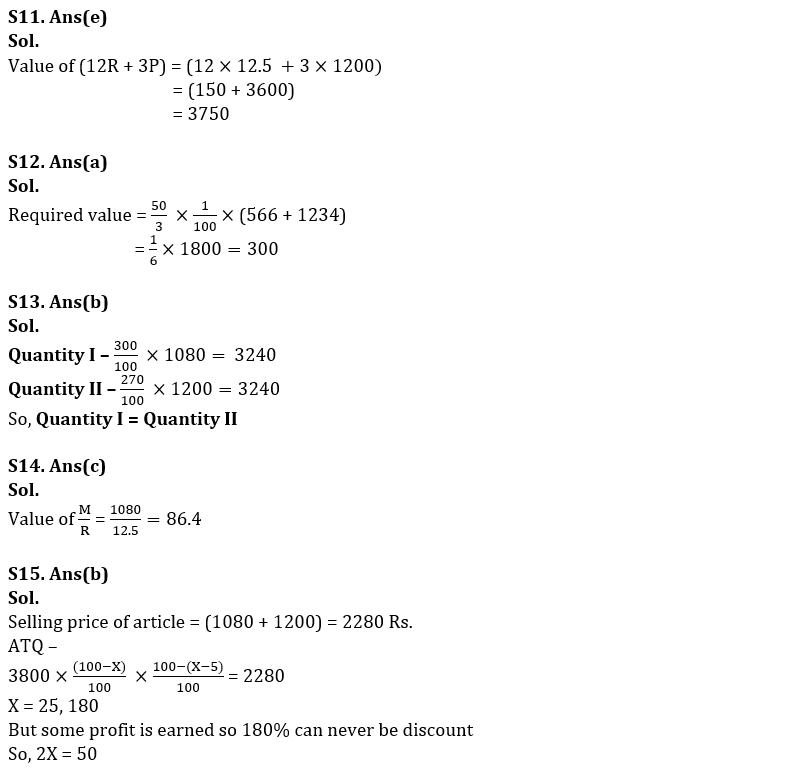#### Congratulations!Union Budget 2023-24: Free PDF